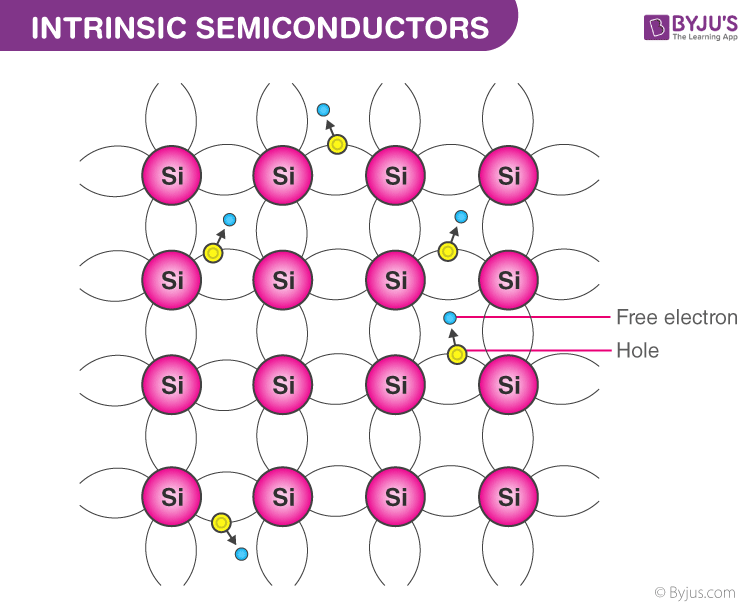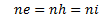# Intrinsic Semiconductors

A semiconductor is a material whose electrical conductivity falls between that of a conductor and an insulator. Based on the level of purity, semiconductors are classified into intrinsic and extrinsic semiconductors. Here in this article, let us discuss the intrinsic semiconductor in detail.

## What are Intrinsic Semiconductors?

Semiconductors that are chemically pure, in other words, free from impurities are termed as intrinsic semiconductors. The number of holes and electrons is therefore determined by the properties of the material itself instead of the impurities. In intrinsic semiconductors, the number of excited electrons is equal to the number of holes; n = p. They are also termed as undoped semiconductors or i-type semiconductors. Silicon and germanium are examples of i-type semiconductors. These elements belong to the IVth Group of the periodic table and their atomic numbers are 14 and 32 respectively.Electrons and Holes configuration in a Silicon atom

### Working Mechanism of Intrinsic Semiconductors

 Electronic Configuration of Silicon and Germanium Silicon 1s2 2s22p6 3s2 3p2 Germanium 1s2 2s2 2p6 3s2 3p6 4s2 3d10 4p2

We notice from the electron configurations of both the elements that they have four electrons in their outermost shell or valence shell. As the temperature of the semiconductor is increased, the electrons gain more thermal energy and thus break free from their shell. The process of ionization of the atoms in the crystal lattice creates a vacancy in the bond between the atoms. The position from which the electron gets dislodged has a hole which is equivalent to an effective positive charge. The hole is then occupied by a free electron, as a result of which the latter vacant position becomes a hole and the former becomes a neutral position. This way the hole or the effective positive charge is transferred from one position to another. In an intrinsic semiconductor, the number of free electrons is equal to the number of holes. Mathematically,Here, the ni gives the number of total intrinsic carrier concentration which is equal to the total number of holes or the total number of electrons.

When the temperature of an intrinsic semiconductor is T=0K, it behaves like an insulator. When the temperature is increased further, (T>0), the electrons get excited and move from the valence band to the conduction band. These electrons occupy the conduction band partially, leaving a correspondingly equal number of holes in the valence band.

Since you are here, you might want to check out the following links:

## Frequently Asked Questions on Intrinsic Semiconductor

Q1

### What are intrinsic semiconductors?

Intrinsic semiconductors are those made from the purest form of semiconductor material.

Q2

### What is used by the intrinsic semiconductors for conduction at room temperature?

Electrons and holes are used.

Q3

### How does the intrinsic semiconductor behave at absolute zero?

At absolute zero, the intrinsic semiconductor behaves like an insulator.

Q4

### What happens when an intrinsic semiconductor is doped with trivalent impurity?

When a trivalent impurity is doped with an intrinsic semiconductor, a p-type semiconductor is obtained.

Q5

### Why n-p-n transistors are preferred over p-n-p transistors?

The mobility of electrons is higher than the mobility of holes in the n-p-n transistor when compared to p-n-p transistor.

Test your knowledge on Intrinsic semiconductors